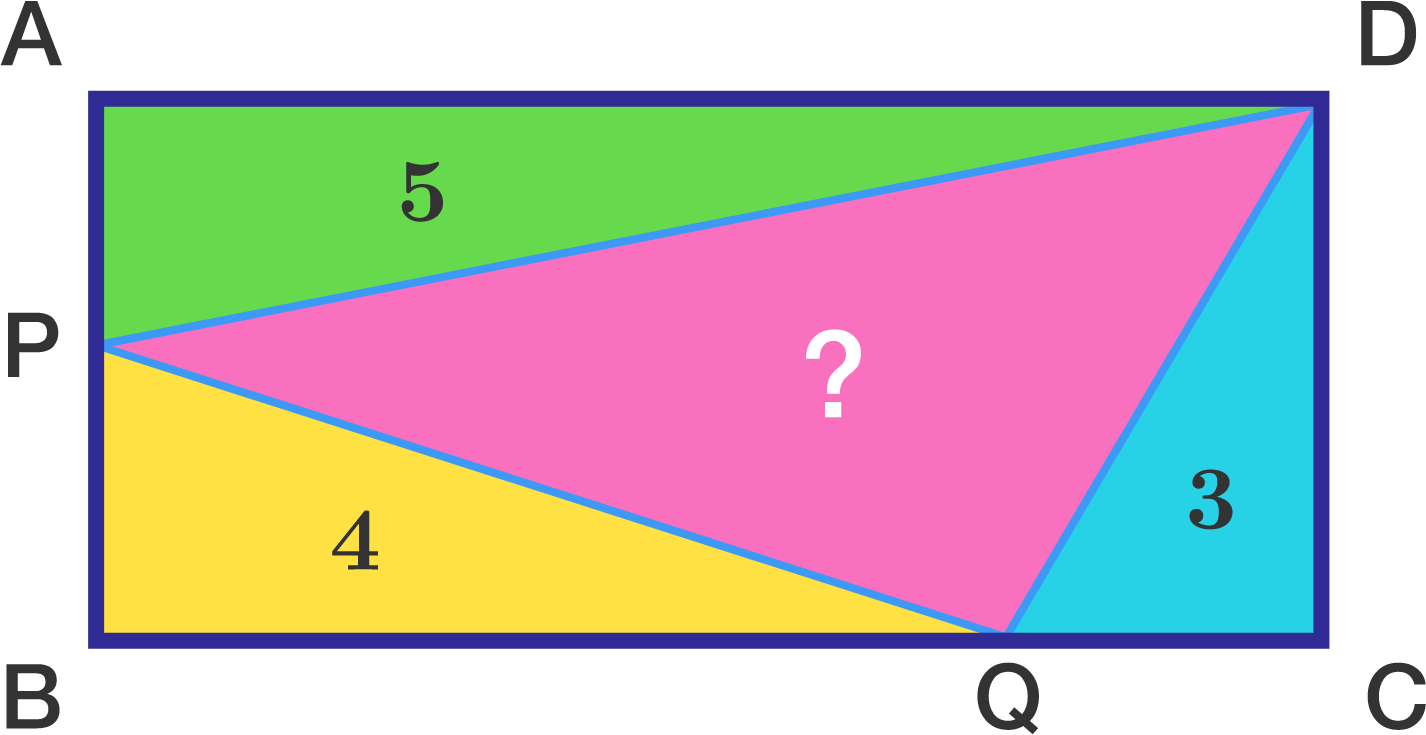# Area of Rectangle

Geometry Level 3

The rectangle below is split into 4 triangles. The areas of these triangles but one are known. What is the area of the unknown triangle $DPQ?$

If this area can be expressed as $\sqrt Q,$ submit your answer as $Q.$×